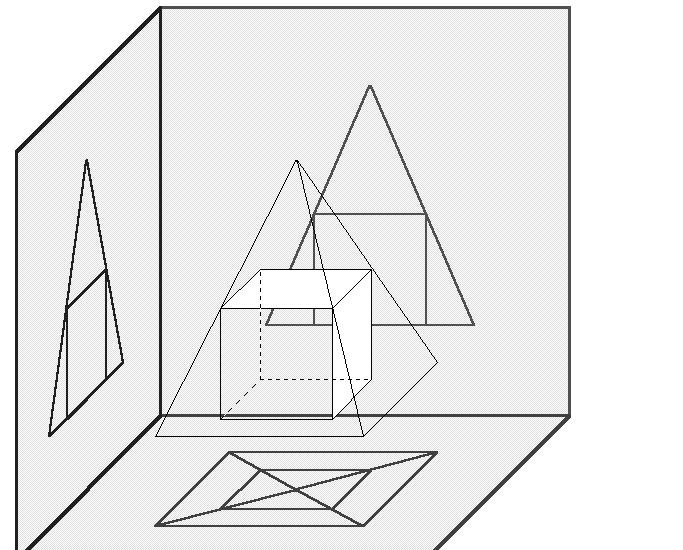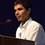# Pyramid questionA pyramid has a square base with sides of length 1 and has lateral faces that are equilateral triangles.

A cube is placed within the pyramid so that one face is on the base of the pyramid and its opposite face has all its edges on the lateral faces of the pyramid.

Find volume of this cube.Note by Piyushkumar Palan
6 years, 10 months ago

This discussion board is a place to discuss our Daily Challenges and the math and science related to those challenges. Explanations are more than just a solution — they should explain the steps and thinking strategies that you used to obtain the solution. Comments should further the discussion of math and science.

When posting on Brilliant:

• Use the emojis to react to an explanation, whether you're congratulating a job well done , or just really confused .
• Ask specific questions about the challenge or the steps in somebody's explanation. Well-posed questions can add a lot to the discussion, but posting "I don't understand!" doesn't help anyone.
• Try to contribute something new to the discussion, whether it is an extension, generalization or other idea related to the challenge.

MarkdownAppears as
*italics* or _italics_ italics
**bold** or __bold__ bold
- bulleted- list
• bulleted
• list
1. numbered2. list
1. numbered
2. list
Note: you must add a full line of space before and after lists for them to show up correctly
paragraph 1paragraph 2

paragraph 1

paragraph 2

[example link](https://brilliant.org)example link
> This is a quote
This is a quote
    # I indented these lines
# 4 spaces, and now they show
# up as a code block.

print "hello world"
# I indented these lines
# 4 spaces, and now they show
# up as a code block.

print "hello world"
MathAppears as
Remember to wrap math in $$ ... $$ or $ ... $ to ensure proper formatting.
2 \times 3 $2 \times 3$
2^{34} $2^{34}$
a_{i-1} $a_{i-1}$
\frac{2}{3} $\frac{2}{3}$
\sqrt{2} $\sqrt{2}$
\sum_{i=1}^3 $\sum_{i=1}^3$
\sin \theta $\sin \theta$
\boxed{123} $\boxed{123}$

Sort by:

Hi, check that a smaller square pyramid is being formed at the top having side same as that of cube (say $s$),

Now, height of pyramid = $\frac{s}{\sqrt{2}}$

Now height from top to bottom = $\frac{1}{\sqrt{2}}$,

Hence,

$s + \frac{s}{\sqrt{2}} = \frac{1}{\sqrt{2}}$

Solve to get volume = $s^3 \approx 0.07106$ cu. unit

- 6 years, 10 months ago

Yes...

thats $\boxed{5\sqrt2 - 7} ..$ thats the answer

Thanks ..

- 6 years, 10 months ago

let the horizontal side of the cube at the top equals x

let the vertical side of the cube equals y

let the height of the pyramid is $h = 1/\sqrt{2}$

now x divides the lateral sides of the pyramid to two lengths ,let the top length equals k and the bottom length M

$x = k$

since the base sides equals lateral sides of the pyramid is 1

Now y divides the lateral side of the cube to two lengths ,M and K

$y/h = M$

$y = M/\sqrt{2}$

$x = y$

then$k = M/\sqrt{2}$

$M+k = 1$

$k + k*\sqrt{2}= 1$

$x/1 = k/1$

$x = k/(k+k*\sqrt{2})$

$x = 1/(1+\sqrt{2})$

- 6 years, 9 months ago

(Need Help)How to find the side of a regular tetrahedron which is inside a sphere of radius ' r ', Exactly fitting? Just as a triangle has circumcircle, say the Tetrahedron has a CIRCUMSPHERE...

- 6 years, 10 months ago

Aditya, i ve moved yours and more of mine questions on 3D geometry in New Discussion

- 6 years, 10 months ago

Thank u very much sir :)

- 6 years, 9 months ago

the answer is 0.0999 cubic units... let me know whether it is correct or not thanks in advance.

- 6 years, 10 months ago

no sunny ... Jatin has got it right

- 6 years, 10 months ago